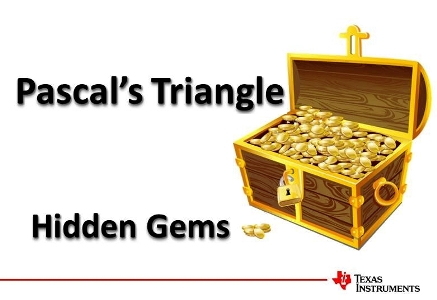# Activities

•• ##### AuthorAust Senior

50 Minutes

• ##### Device
•TI-84 Plus CE

## Pascals Triangle Hidden Gem#### Activity Overview

Pascal's Triangle goes way beyond coefficients of binominal expansions and combinatorics. In this activity students are introduced to the basics but go on to explore other relationships and patterns such as the triangular and tetrahedral numbers, Fibonacci sequence and even Euler's number. There are so many hidden gems in this amazing triangle.

#### Objectives

By exploring Pascal’s triangle in greater depth, students are more likely to remember this amazing triangle. The numeric and algebraic applications will help improve students ‘by-hand’ algebraic skills beyond basic simplification.

#### Vocabulary

• Pascal
• Combinatorics
• Binomial Expansion
• Triangular Numbers
• Tetrahedral Numbers
• Fibonacci
• Euler